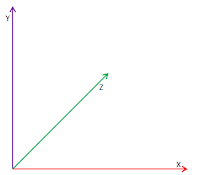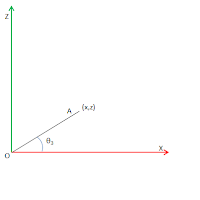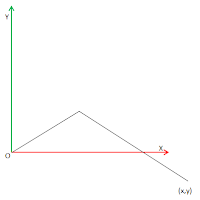Saturday, 26 January 2013

Hexapod Inverse Kinematics - Part 2

Part 1 covered how to perform inverse kinematics when the destination of the Phoenix Hexapod foot is specified in the X and Y axis only. This post will cover how to perform inverse kinematics when the foot position is specified in the X, Y and Z axis. The cartesian co-ordinate system used for the analysis is shown in figure 1 below.Figure 1: Catesian co-ordinate system
Consider the situation where the foot is to be placed at a position A defined by (x,y,z). Figure 2 shows the top down view in the X,Z axis with a side on view along the OA plane in the X,Y axis shown in figure 3.Figure2: top down view in X,Z axisFigure 3: side on view along OA plane in the X,Y axis
Angle θ3 (the coxa angle) can easily calculated from the X and Z co-ordiantes. Next rotate the leg by θ3 degrees such that Z is 0. This will rotate the OA plane such that it is aligned with the XY plane. The femur and tibia angles can be calculated as per the Part 1. Please note that the rotation remaps the x co-ordinate of the foot. In summary for a desired foot location of (x,y,z)
the location of the foot in the XY axis after the rotation will be (x1, y).
Unfortunately there is a slight complication due to the design of the hexapod as shown in figures 4 and 5.Figure 4: Top down view of legFigure 5: Compensating for actual pivot in XZ axis
Till now all the analysis assumed that the hexapod leg effectively rotated around the femur in the XZ plane. This assumption is not correct as evident from figure 4 due to the distance of 29mm between the actual pivot (coxa servo) and the femur servo. Figure 5 shows that the location of the femur is not fixed and infact changes as the coxa servo rotates. To study the impact of this offset consider point C which is 80mm from point B. To simplify the analysis the Y axis will be ignored. The leg will now be moved to point D such that angle DBC = 26.57 degrees. If the coxa was directed to rotate by 26.57 degree the foot will end up at location E. To compensate have the coxa servo rotate by angle DAC instead of DBC. Please note this analysis was based on the assumption that vector BC cuts through the centre of rotation point of the coxa when the coxa servo is at 0 degrees. This is not true and the vector BC is infact slightly away from the depiction in figure 5. I am unable to compensate for this factor without a detailed model of the leg to measure the actual distance between the actual points. However I am open to suggestions.